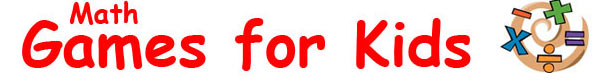Enjoy an awesome range of fun math games for kids. Learn about important mathematics topics such as arithmetic, geometry, numbers and statistics while improving your problem solving skills and math basics online. Put your math skills to the test by completing interactive activities, solving equations and conquering fun challenges. Practice your addition and multiplication skills, test how many shapes you know, experiment with probability, understand how number patterns work, learn about fractions and much more. Have fun learning about a variety of math topics with these free games for kids. Choose one you like the look of and give it a go! Sponsored Links

 Sponsored LinksArithmetic Games
 Work on your math basics with these great arithmetic games for kids. Use addition, subtraction, multiplication and division to answer a range of arithmetic based questions. Complete as many of the challenges as you can and have fun improving your math skills.
 Addition Have fun building a giant water slide while working on your addition skills with this interactive math game for kids.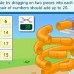Subtraction Make calculations and use your subtraction skills to help cut the right sized pieces of pipe for an awesome water slide.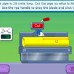Multiplication Solve a range of multiplication problems with this fun activity that's perfect for kids. Can you solve them all? Give it a try and find out!Division Work on your division skills with this interactive game. Watch the flashing signs before clicking on the correct answer.Geometry Games
 Improve your geometry skills with these games and activities for kids. Learn about shapes such as triangles, squares and circles, measure the length and weight of packages, find locations and coordinates, use angles to squirt objects with a water hose and more.
 Shapes Learn about geometric and 3D shapes while completing a range of cool challenges involving quadrilaterals, triangles and more.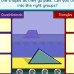Angles Rotate the water hose and squirt various objects while learning about angles with this cool game for kids. How many targets can you hit?Measurements Use interactive scales and a ruler to measure different shaped parcels with this fun measurement game for kids.Grids Learn about grids and coordinates with this interactive math activity. Use a compass to move your car in the right direction.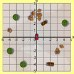Transformations Learn the concepts of rotation, reflection and translation with this great game. Complete the challenges and have fun!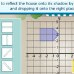Number Games
 Have fun learning about numbers with these awesome games and activities for kids. Learn how to use fractions and decimals, improve your problem solving skills, practice shopping with money, understand how to use a computer and complete a range of math equations.
 Fractions Put fractions in their correct order, cut pipes into the correct lengths and complete a range of challenges with this educational game.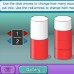Decimals Examine a range of decimal frames and try putting them in the right order with this interactive math game for kids.Number Patterns Move numbers into their correct positions, solve the problems, crack the code and unlock the safe with this cool number game for kids.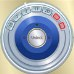Place Values Learn about tens, hundreds and thousands, complete simple equations, and enjoy fun activities while learning how place values work.Calculators This excellent learning activity helps kids learn how to use calculators while using the various functions to solve fun number problems.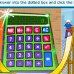Shopping with Money Learn how to keep track of money while completing a range of shopping based challenges with this interactive game for kids.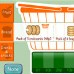Problem Solving Train your brain to do mental math, learn how to handle numbers and improve your problem solving skills with this fun math game.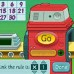Statistics Games
 Enjoy these interactive math games that will help improve kids understanding of statistics topics. Experiment with a probability machine, plan a park using the right resources, make a frequency table and learn about the mean, median and mode of data sets.
 Probability Learn about probability with this fun game for kids. What kind of results will you get from the random ball machine? Give it a try and find out!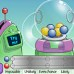Percentages Learn about percentages while planning a park with the right amount of grass, water, woodland, flowers and playgrounds.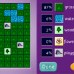Mean, Median & Mode Try this fun math game for kids and learn about the concepts of mean, median and mode while calculating values from different data sets.Frequency Tables Tally the results of a survey and turn the information into useful tables, charts and graphs with this educational math game for kids.Science Kids ©  |  Home  |  About  |  Topics  |  Experiments  |  Games  |  Facts  |  Quizzes  |  Projects  |  Lessons  |  Images  |  Videos  |  Privacy  |  Sitemap  |  Updated: Jan 14, 2021